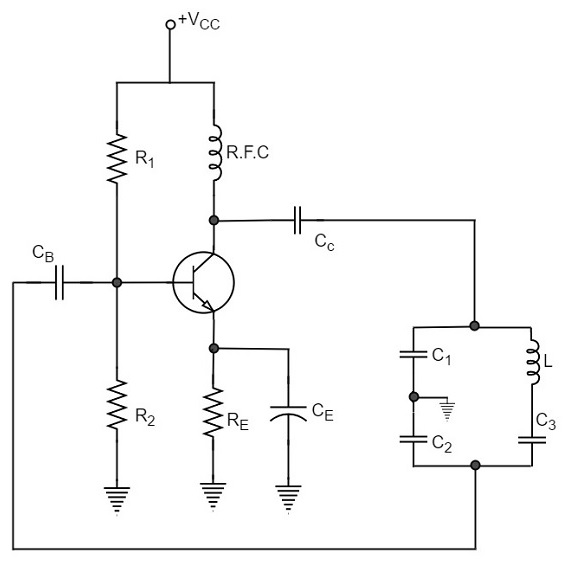# Clapp Oscillator

Another oscillator which is an advanced version of Colpitts oscillator is the Clapp Oscillator. This circuit is designed by making a few changes to the Colpitts oscillator.

The circuit differs from the Colpitts oscillator only in one respect; it contains one additional capacitor (C3) connected in series with the inductor. The addition of capacitor (C3) improves the frequency stability and eliminates the effect of transistor parameters and stray capacitances.

The following circuit diagram shows the arrangement of a transistor Clapp oscillator.The operation of Clapp oscillator circuit is in the same way as that of Colpitts oscillator. The frequency of oscillator is given by the relation,

$$f_o = \frac{1}{2 \pi \sqrt{L.C}}$$

Where

$$C = \frac{1}{\frac{1}{C_1} + \frac{1}{C_2} + \frac{1}{C_3}}$$

Usually, the value of C3 is much smaller than C1 and C2. As a result of this, C is approximately equal to C3. Therefore, the frequency of oscillation,

$$f_o = \frac{1}{2 \pi \sqrt{L.C_3}}$$

It is understood that the Clapp oscillator is similar to the Colpitts oscillator, however they differ in the way the inductances and capacitances are arranged. The frequency stability though is good, can be variable in a Clapp oscillator.

A Clapp oscillator is sometimes preferred over a Colpitts oscillator for constructing a variable frequency oscillator. The Clapp oscillators are used in receiver tuning circuits as a frequency oscillator.